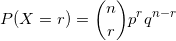# I. Determine whether each of t

I. Determine whether each of the following random variables hasa binomial distribution. If it does, identify the values of theparameters n and p. If not, explain.

a) X is the number of times five is rolled in 15 rolls of afair, six-sided die.

b) X is the number of multiple-choice questions a student getsright on a 30-question test, when each question has four choicesand the student is completely guessing on each question.

c) X is the same as (b), but the first 15 questions have fourchoices and the last 15 have three answer choices.

d) X is the number of women in a random sample of 12 students,from a class comprising 25 women and 20 men.

e) X is the total weight of 16 randomly selected oranges

f) X is the number of oranges, out of a random sample of 16,that weight more than 170 grams

Binomial Distribution

If ‘X’ is the randomvariable representing the number of successes, the probability ofgetting ‘r’ successes and‘n-r’failures, in ‘n’ trails,‘p’probability of success ‘q’=(1-p) is givenby the probability functiona.X is the number of times five is rolled in 15 rolls of a fair,six-sided die.

Success : Five is rolled

X :Number of successes: Number of times five is rolled:

n : Number of trails : 15 rolls of a fair die

p: Probability of success : Probability of rolling a five=1/6

Hence ‘X’ has a binomial distribution with n=15 and p=1/6

b. X is the number of multiple-choice questions a student getsright on a 30-question test, when each question has four choicesand the student is completely guessing on each question.

Success : questions a student gets right

X :Number of successes: number of multiple-choice questions astudent gets right

n : Number of trails : 30-question test

p: Probability of success : Probability of getting a questionright = 1/4 (As number of choices is 4)

Hence ‘X’ has a binomial distribution with n= 30 and p=1/4

c) X is the same as (b), but the first 15 questions have fourchoices and the last 15 have three answer choices

p : Probability of success : Probability of getting a questionright for the first 15 questions= 1/4 (As number of choices is 4)and : Probability of getting a question right for the last 15questions= 1/3 (As number of choices is 3)

As ‘p’: probability is not same across the trails , ‘X’ does notfollow Binomial distribution.

d)

X is the number of women in a random sample of 12 students, froma class comprising 25 women and 20 men

Success : Having a women in the random sample

X :Number of successes:   number of women in a randomsample

n : Number of trails : sample size of the random sample = 12

p: Probability of success : Probability of selecting a women =Number of women in the class / Total number of (women+Men) =25/25+20=25/45 = 5/9

Hence ‘X’ has a binomial distribution with n=12 and p=5/9

e)

X is the total weight of 16 randomly selected oranges

X : Number of successes ;

As X : Total weight can not be defined as a success;

‘X’ does not follow Binomial distribution.

f)  X is the number of oranges, out of a random sampleof 16, that weight more than 170 grams

Success : Orange that weight more than 170 grams

X : Number of success : Number of oranges whose weight more than170 grams

n : number of trails = Number of oranges in the random sample =16

p: Probability that an orange weighs more than 170 grams.(Notknown)

Therefore ‘X’ has a binomial distribution with n= 12 and p :Probability that an orange weighs more than 170 grams: notknown

##### "Our Prices Start at \$11.99. As Our First Client, Use Coupon Code GET15 to claim 15% Discount This Month!!"Calculate the price
Pages (550 words)
\$0.00
*Price with a welcome 15% discount applied.
Pro tip: If you want to save more money and pay the lowest price, you need to set a more extended deadline.
We know how difficult it is to be a student these days. That's why our prices are one of the most affordable on the market, and there are no hidden fees.

Instead, we offer bonuses, discounts, and free services to make your experience outstanding.
How it works
Receive a 100% original paper that will pass Turnitin from a top essay writing service
step 1
Fill out the order form and provide paper details. You can even attach screenshots or add additional instructions later. If something is not clear or missing, the writer will contact you for clarification.
Pro service tips
How to get the most out of your experience with brilliantassignmenthelp.com
One writer throughout the entire course
If you like the writer, you can hire them again. Just copy & paste their ID on the order form ("Preferred Writer's ID" field). This way, your vocabulary will be uniform, and the writer will be aware of your needs.
The same paper from different writers
You can order essay or any other work from two different writers to choose the best one or give another version to a friend. This can be done through the add-on "Same paper from another writer."
Copy of sources used by the writer
Our college essay writers work with ScienceDirect and other databases. They can send you articles or materials used in PDF or through screenshots. Just tick the "Copy of sources" field on the order form.
Testimonials
See why 20k+ students have chosen us as their sole writing assistance provider
Check out the latest reviews and opinions submitted by real customers worldwide and make an informed decision.
11,595
Customer reviews in total
96%
Current satisfaction rate
3 pages
Average paper length
37%
Customers referred by a friendUse a coupon FIRST15 and enjoy expert help with any task at the most affordable price.
Claim my 15% OFF Order in Chat